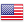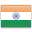Grade "A+" Accredited by NAAC with a CGPA of 3.46
Grade "A+" Accredited by NAAC with a CGPA of 3.46

# Introduction to R Programming

Course ID
BHCS 20B
Level
Program
B.Sc. CS (Hons.)
Semester
Fourth
Credits
4.0
Paper Type
Skill Enhancement - 2
Method
Lecture & Practical

## Unique Paper Code: Update Awaited

This course introduces R, which is a popular statistical programming language. The course covers data reading and its manipulation using R, which is widely used for data analysis internationally. The course also covers different control structures and design of user-defined functions. Loading, installing and building packages are covered.

## Learning Outcomes:

At the end of the course, students should be able to:

• Develop an R script and execute it.
• Install, load and deploy the required packages, and build new packages for sharing and reusability.
• Extract data from different sources using API and use it for data analysis.
• Visualize and summarize the data.
• Design application with database connectivity for data analysis.

## Course Contents

Unit 1
Unit 2
Unit 3
Unit 4

Unit 1

Introduction: R interpreter, Introduction to major R data structures like vectors, matrices, arrays, list and data frames, Control Structures, vectorized if and multiple selection, functions.

Unit 2

Installing, loading and using packages: Read/write data from/in files, extracting data from web-sites, Clean data, Transform data by sorting, adding/removing new/existing columns, centring, scaling and normalizing the data values, converting types of values, using string in-built functions, Statistical analysis of data for summarizing and understanding data, Visualizing data using scatter plot, line plot, bar chart, histogram and box plot.

Unit 3

Designing GUI: Building interactive application and connecting it with database.

Unit 4

Building Packages.

### Practicals

#### Lab List 1

1. Write an R script to do the following:
a) simulate a sample of 100 random data points from a normal distribution with mean 100 and standard deviation 5 and store the result in a vector.
b) visualize the vector created above using different plots.
c) test the hypothesis that the mean equals 100.
d) use wilcox test to test the hypothesis that mean equals 90.
2. Using the Algae data set from package DMwR to complete the following tasks.
a) create a graph that you find adequate to show the distribution of the values of algae a6.
b) show the distribution of the values of size 3.
c) check visually if oPO4 follows a normal distribution.
d) produce a graph that allows you to understand how the values of NO3 are distributed across the sizes of river.
e) using a graph check if the distribution of algae a1 varies with the speed of the river.
f) visualize the relationship between the frequencies of algae a1 and a6. Give the appropriate graph title, x-axis and y-axis title.
3. Read the file Coweeta.CSV and write an R script to do the following:
a) count the number of observations per species.
b) take a subset of the data including only those species with at least 10 observations.
c) make a scatter plot of biomass versus height, with the symbol colour varying by species, and use filled squares for the symbols. Also add a title to the plot, in italics.
d) log-transform biomass, and redraw the plot.
4. The built-in data set mammals contain data on body weight versus brain weight. Write R commands to:
a) Find the Pearson and Spearman correlation coefficients. Are they similar?
b) Plot the data using the plot command .
c) Plot the logarithm (log) of each variable and see if that makes a difference.
5. In the library MASS is a dataset UScereal which contains information about popular breakfast cereals. Attach the data set and use different kinds of plots to investigate the following relationships:
a) relationship between manufacturer and shelf
b) relationship between fat and vitamins
c) relationship between fat and shelf
d) relationship between carbohydrates and sugars
e) relationship between fibre and manufacturer
f) relationship between sodium and sugars
6. Write R script to:
a) Do two simulations of a binomial number with n = 100 and p = .5. Do you get the same results each time? What is different? What is similar?
b) Do a simulation of the normal two times. Once with n = 10, µ = 10 and σ = 10, the other with n = 10, µ = 100 and σ = 100. How are they different? How are they similar? Are both approximately normal?
7. Create a database medicines that contains the details about medicines such as {manufacturer, composition, price}. Create an interactive application using which the user can find an alternative to a given medicine with the same composition.
8. Create a database songs that contains the fields {song_name, mood, online_link_play_song}. Create an application where the mood of the user is given as input and the list of songs corresponding to that mood appears as the output. The user can listen to any song form the list via the online link given.
9. Create a package in R to perform certain basic statistics functions.
Mini project using data set of your choice from Open Data Portal (https://data.gov.in/) for the following exercises

#### Text Books

Cotton, R., Learning R: a step by step function guide to data analysis. 1st edition. O’reilly Media Inc

Gardener, M.(2017). Beginning R: The statistical programming language, WILEY
Lawrence, M., & Verzani, J. (2016). Programming Graphical User Interfaces in R. CRC press. (ebook)

#### Web Resources

https://jrnold.github.io/r4ds-exercise-solutions/index.html
https://www.r-project.org/
https://cran.r-project.org/

#### Teaching Learning Process

Use of ICT tools in conjunction with traditional class room teaching methods
Interactive sessions
Class discussions

#### Assessment Methods

Written tests, assignments, quizzes, presentations as announced by the instructor in the class

#### Keywords

R data structures, flow control, packages, functions.

Disclaimer: Details on this page are subject to change as per University of Delhi guidelines. For latest update in this regard please refer to the University of Delhi website here.Englishहिन्दी